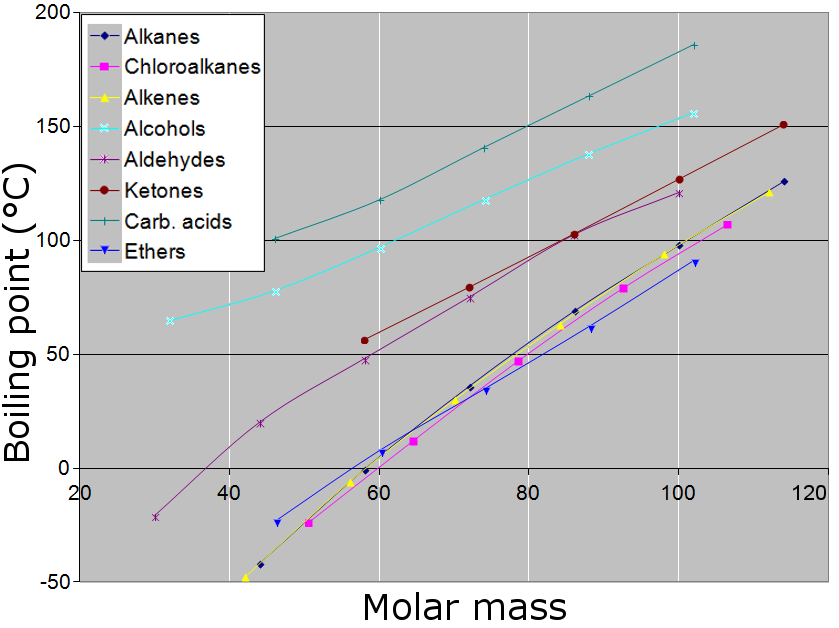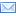#Difference Between Similar Terms and Objects

# Difference between Molar Mass and Molecular Mass

Chemists frequently measure substances by using molar mass and molecular mass. An example would be determining the number of molecules in a cylinder. Chemistry studies the interaction of atoms and molecules on an atomic scale.## What is a Molecular Mass?

Firstly, molecule refers to the composition of similar or different atoms arranged in ratios. The molecules can form chemical compounds subject to the physical and chemical properties of each molecule.

In a nutshell, a molecular mass refers to the total mass of a substance. It sums the average masses of individual atoms in a molecule of that particular substance.

In order to determine the molecular mass of a compound X, you have to add the atomic masses of all the elements in the composition including their multiplications as per the subscripts in the formula.

We will illustrate with an example below. These atomic masses are taken directly from the periodic table of elements. They are measured in atomic mass units (amu) and so is the molecular mass.

Example 1:

Determine the molecular mass of H2O and CH3CH2OH.

You have to assess the compositions of the given molecular formulas and derive their atomic masses from the periodic table. Take into account the subscripts.

For example, H2O indicates that there are two hydrogen atoms and one oxygen atom. The atomic mass of Hydrogen is, from the periodic table, 1.0079 amu and that of oxygen is 15.9994. The molecular mass of the compound is 2(1.0079) + 15.9994 = 18.0152 amu.

And to determine the molecular mass of ethanol, the other formula given in the question, we follow the same steps. There are 2 Carbon atoms, 6 Hydrogen atoms and 1 Oxygen atom. The atomic mass of Carbon atom is 12.011 amu. That said, the molecular mass of CH3CH2OH is 2(12.011) + 6(1.0079) + 1(15.9994) = 46.069 amu. For your exercise, calculate the molecular formula of ethane (C2H4) and CO2.## What is Molar Mass?

Now regarding the molar mass concept, it is defined as the mass in kilograms or grams of a mole of a substance. Usually, the units for molar mass are gram/mol. A mole of an oxygen element has a mass of 15.9994 grams. Thus the molar mass would be 15.9994 g/mol. For a carbon dioxide compound, for example, the molar mass is defined as the mass of 1 mole of molecules in that compound. Avogadro’s number, 6.022 x 1023 is often used to relate the number of atoms, molecules and other units that a molar mass is equal to. For instance, a molar mass of H2O would be equal to the mass in grams per mole of 6.022 x 1023 water molecules. If this sounds too technical, we will illustrate with an example below:

Example 2:

Determine the molar mass of ethanol.

In the first example, we determined the molecular mass of ethanol (C2H5OH). Don’t be confused by a different formula as the aforementioned. We have just played around with the structural arrangements of ethanol formula although the ratio of atoms remains the same.

Ethanol contains 6.022 x 1023molecules, and the molar mass is equal to the mass of ethanol that contains these molecules. We found the molecular mass of ethanol to be 46.069 atomic mass units in our previous example. The same procedure is followed in calculating the molar mass.

One mole of ethanol has 2 mole Carbon atoms, 6 mole of Hydrogen atoms and one mole of Oxygen atom. Taking the atomic masses from the period table, we find that the molar mass is 2(12.011g) + 6(1.0079g) + 15.9994g which is equal to 46.069 g/mo. Notice the same value but different units.

NB: The molar mass of any chemical substance refers to its atomic mass or molecular mass in grams per mole. The values are the same but different units.

Exercise 2:

Calculate the molar mass of Calcium Phosphate (Ca3(PO4)2). The answer should be 310.177 g/mol.

With regard to carbon, the molar mass of a naturally occurring carbon with be different from the one derived from the period table which belongs to carbon-12 isotope. Naturally, carbon contains carbon-12, carbon-13 and carbon-14. But a mole of carbon still contains 6.022 x 1023 but with different ratios of these isotopes.

## Differences between Molar Mass and Molecular Mass

### Calculation for Molar Mass and Molecular Mass

Molar mass is determined by dividing the total mass of the substance which is the average masses of each atom by the amount in moles. The resulting figure will be grams per mole or kilograms per mole. The molecular mass is determined by calculating the mass of the substance as related to the 1/12th of carbon-12 isotope.

### Definition of Molar Mass and Molecular Mass

Molecular mass refers to the mass of the molecules whereas Molar mass refers to the mass of a mole of a substance.

### SI Units for Molar Mass and Molecular Mass

Molar mass is expressed as the grams per mole whereas a molecular mass is expressed as atomic mass units. This expression of SI units goes back to how these concepts are defined as aforementioned.## Summary of Molar Mass Vs. Molecular Mass

• Molar mass and molecular mass are important concepts in chemistry
• Molar mass is expressed in grams per mole or kilograms per mole whereas molecular mass is expressed in atomic mass units. Molar mass is basically unitless.
• We calculate molar mass by dividing the mass of the substance by the amount and calculate the molecular mass by taking average mass of the units of the substance
• They have the same values but different SI units.

### Search DifferenceBetween.net :

Custom Search

Help us improve. Rate this post!Loading...Email This Post : If you like this article or our site. Please spread the word. Share it with your friends/family.

1. Thank you so much for the detailed explanation. Now only I understood that both have the same magnitude but differ in their sign and defn only.

2. Sir,
please reply me whether I am correct or not.
Molecular mass of CO is 27.9994 a.m.u and the Molar mass of CO is 27.9994 g/mol.

Please note: comment moderation is enabled and may delay your comment. There is no need to resubmit your comment.

## References :